## Want to keep learning?

This content is taken from the Raspberry Pi Foundation & National Centre for Computing Education's online course, How Computers Work: Demystifying Computation. Join the course to learn more.
1.8

## Raspberry Pi Foundation

Skip to 0 minutes and 1 second In this step, we’re going to look at working with binary. As you saw in a previous step, a Turing machine is capable of operating using only two symbols, 1 and 0. And the rather bold statement was made that using just these symbols, any solvable problem could be solved. How can this be? How can you even add two numbers, say 12 and 6, if you only have the ability to use ones and zeros? To answer this question, we should take a brief look at the nature of numbers. When you were learning to do basic arithmetic when you were a child, you probably learned about counting in ones, tens, hundreds, and thousands. This is called base 10, or denary.

Skip to 0 minutes and 45 seconds When writing down numbers, you begin at 0, count all the way up to 9, then go back to 0 again, but have an additional digit in the next column. You could ask yourself, why is it that we have 10 separate symbols to represent all our numbers? Why not five? Then counting would look like this. The answer is most probably because we have 10 fingers and thumbs, although it’s not really known. Some cultures around the world count in different bases, and most people are fairly happy using base 12 and base 60 when telling the time. The Turing machine uses base 2, or what is often called binary. Here’s an example of counting in binary.

Skip to 1 minute and 31 seconds You might recall that this is exactly what your Turing machine did in the last step. So to answer the original question, how could a Turing machine add the numbers 12 and 6, the answer is that the machine would use binary representations of these numbers. Here’s an example of simultaneously counting in both binary and denary. So how can we convert from one to the other? We said earlier that when you were learning about numbers as a child, you probably used the terms ones, tens, hundreds, and thousands. These are all powers of 10. You can draw a table using all these values as headings.

Skip to 2 minutes and 12 seconds You can write in a number, in this case 09032, and we can see that it is made up of zero 10,000s, nine 1,000s zero 100s, three 10s, and two 1s. In binary, we can do the same thing, but using powers of 2.

Skip to 2 minutes and 35 seconds Again, a table can be drawn up using these numbers as headings.

Skip to 2 minutes and 40 seconds As we did with denary, we can write in a binary number, such as 10111. Now we can see it is made up of one 16, zero 8s, one 4, one 2, and one 1. So if this was in denary, it would be the number 23. You’re now going to have a go at doing some binary to denary conversions yourself. So you might like to replay this video and have a look over it again. Pause the video here and have a practise on the following binary numbers, and see if you can work out what they would be in denary. There’ll be a quiz on this in the next section. Converting numbers back the other way isn’t much more difficult.

Skip to 3 minutes and 23 seconds If we wanted to convert the number 21 into binary, we could start out with an empty table like this. Then look in each of the columns to see what needs to be placed there using only ones and zeros. In this case, we would need one 16, zero 8s, one 4, zero 2s, and one 1.

Skip to 3 minutes and 54 seconds Reading off from the table, we can see that 21 in binary is 10101. Have a go with converting the following denary numbers into binary. Don’t forget to share your answers and help each other out in the comments section.

# Working with binary

In this step we’re going to look at working with binary.

As you saw in a previous step, a Turing machine is capable of operating using only two symbols – 1 and 0 – and the rather bold statement was made that, using just these symbols, it can do any computation!

How can this be? How can you even add up two numbers, say 12 and 6, if you only have the ability to use 1s and 0s?

To answer this question, we should first take a brief look at the nature of numbers. When you were learning to do basic arithmetic, when you were a child, you probably learned about counting in ones, tens, hundreds and thousands.

This is called base 10. When writing down numbers, you begin at 0, count up to 9, then go back to 0 again, but add an additional digit in the next column.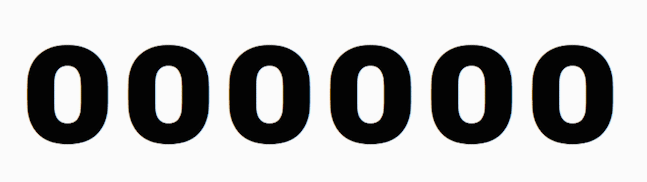You could ask yourself, why is it we have 10 separate symbols to represent all our numbers? Why not 5? Then counting would look like this: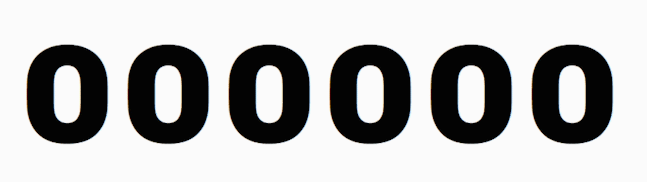The answer is most probably because we have 10 fingers and thumbs, although it’s not really known. Some cultures around the world count in different bases, and most people in the world are fairly happy using base 12 and base 60 when telling the time.

The Turing machine uses base 2, or what is often called binary. Here’s an example of counting in binary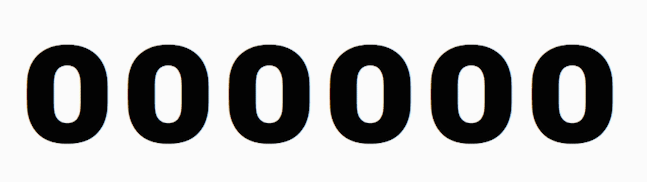You might recall that this is exactly what your Turing machine did in the last step.

So to answer the original question – how could a Turing machine add the numbers 12 and 6? – the answer is that the machine would use the binary representations of these numbers.

## Converting binary to denary

Here’s an example of simultaneously counting in both binary and denary.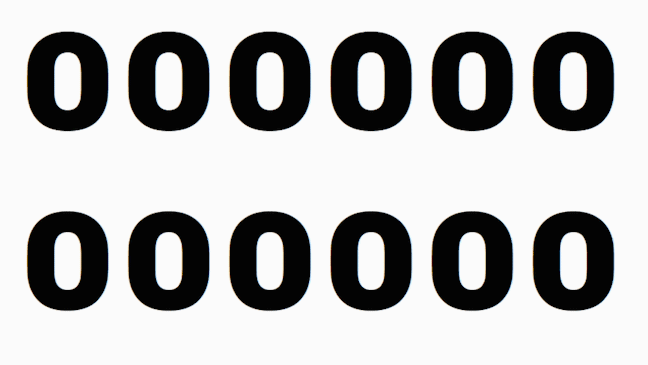So how can we convert from one to another?

We said earlier that when you were learning about numbers as a child, you probably used the terms ones, tens, hundreds, thousands. These are all powers of 10.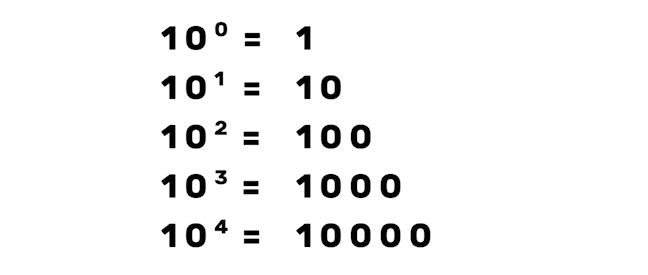We can then arrange these values horizontally to be used as headings.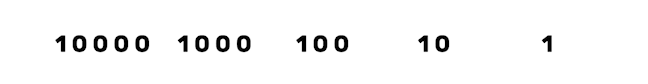Now we can write in a number – in this case 09032 – and we can see that it is made up of 0 x 10000, 9 x 1000, 0 x 100, 3 x 10 and 2 x 1: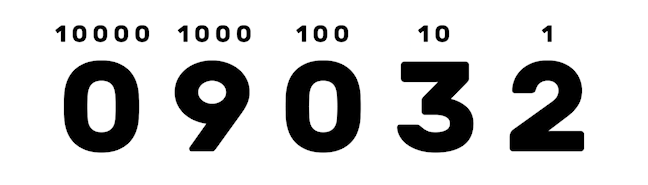In binary we can do the same thing, but using powers of 2.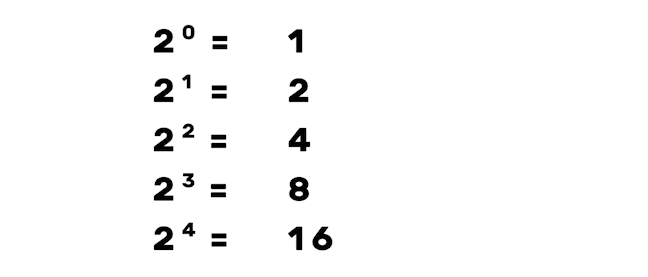Again, a the values are arranged as headings.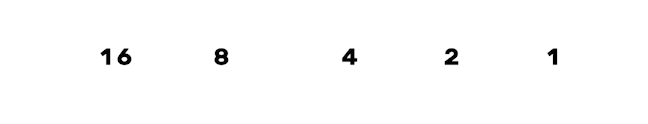As we did with denary, we can write in a binary number such as 10111.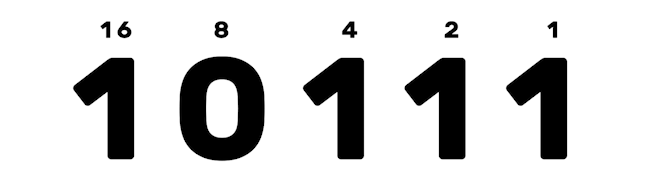Now we can see it is made up of 1 x 16, 0 x 8, 1 x 4, 1 x 2 and 1 x 1. So if this was in denary it would be the number 23.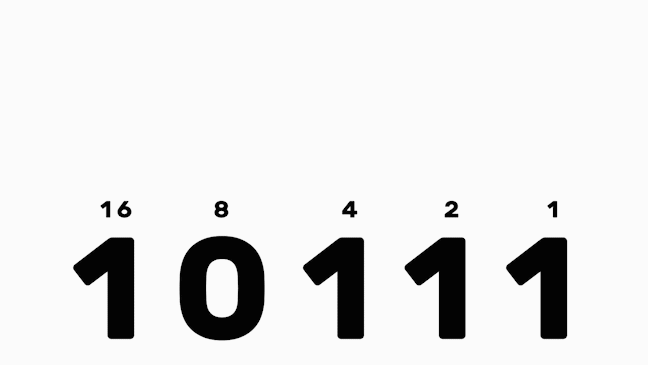Have a practice on the following binary numbers and see if you can work out what they would be in denary. There’ll be a quiz on this in the next section.

1010
1001
10001


Converting numbers back the other way isn’t much more difficult. If we wanted to convert the number 21 into binary we would do it like this: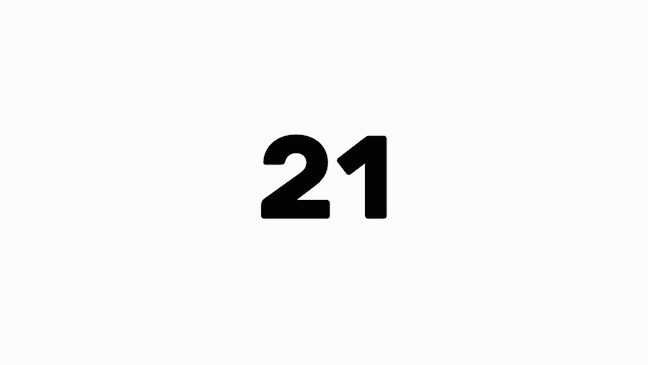We keep dividing the number by a power of two, and then using the remainder for the next division.

Reading off from the table, we can see that 21 in binary is 10101.

Have a go at converting the following denary numbers into binary.

7
15
40


You can compare your answers in the comments section, or even challenge each other to do more binary to denary and denary to binary calculations.